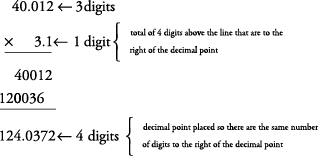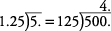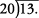## Decimal Computation

You should become efficient in using the four basic operations involving decimals—addition, subtraction, multiplication, and division.

To add or subtract decimals, just line up the decimal points and then add or subtract in the same manner you would add or subtract whole numbers.

##### Example 1

Add 23.6 + 1.75 + 300.002.

Adding in zeros can make the problem easier to work.##### Example 2

Subtract 54.26 – 1.1.##### Example 3

Subtract 78.9 – 37.43.A whole number has an understood decimal point to its right.

##### Example 4

Subtract 17 – 8.43.#### Multiplying decimals

To multiply decimals, just multiply as usual. Then count the total number of digits above the line that are to the right of all decimal points. Place your decimal point in your answer so there are the same number of digits to the right of it as there are above the line.

##### Example 5

Multiply 40.012 × 3.1.#### Dividing decimals

Dividing decimals is the same as dividing other numbers, except that if the divisor (the number you're dividing by) has a decimal, move it to the right as many places as necessary until it is a whole number. Then move the decimal point in the dividend (the number being divided into) the same number of places. Sometimes, you may have to add zeros to the dividend (the number inside the division bracket). Note the decimal point in the quotient (answer) is placed above the one in the dividend.

##### Example 6

Divide##### Example 7

Divide##### Example 8

Divide